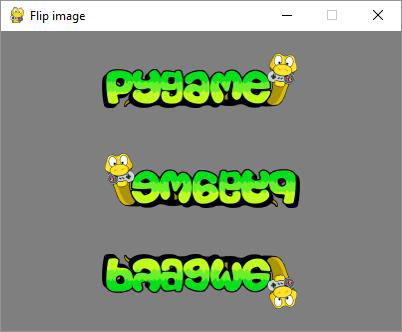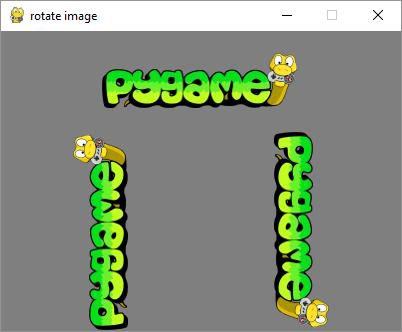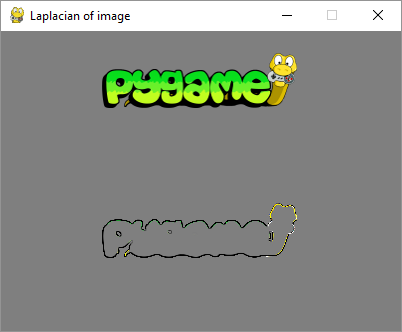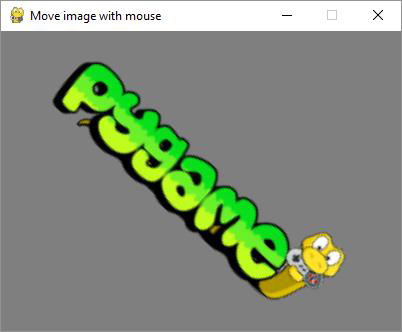# Pygame - Transforming Images

The pygame.ransform module contains definitions of a number of functions for manipulation of Surface objects obtained out of image or text blocks. Manipulation of a surface include flipping, rotation, scaling, resizing and zooming the object.

Following functions are found in pygame.transform module.

 flip() flip vertically and horizontally scale() resize to new resolution rotate() rotate an image rotozoom() filtered scale and rotation scale2x() specialized image doubler smoothscale() scale a surface to an arbitrary size smoothly get_smoothscale_backend() return smoothscale filter version in use - 'GENERIC', 'MMX', or 'SSE' set_smoothscale_backend() set smoothscale filter version to one of - 'GENERIC', 'MMX', or 'SSE' chop() gets a copy of an image with an interior area removed laplacian() find edges in a surface average_surfaces() find the average surface from many surfaces. average_color() finds the average color of a surface threshold() finds which, and how many pixels in a surface are within a threshold of a 'search_color' or a 'search_surf'.

Let us first use the flip() function whose syntax is as follows −

flip(Surface, xbool, ybool)


This function can flip the surface object either horizontally, vertically or both. The orientation is decided by two bool parameters.

To flip the image horizontally, use the following command −

pygame.transform.flip(img2,True, False)


To flip vertically, use the following command −

pygame.transform.flip(img2,False, True)


In the following example, pygame logo image is displayed normally and flipping in both directions. First obtained flipped surface from original image object, fetch its Rect object and then built it. To render horizontally flipped image,

img1 = pygame.image.load('pygame.png')
img2=img1
img2=pygame.transform.flip(img2,True, False)
#inside event loop
rect2 = img2.get_rect()
rect2.center = 200, 150
screen.blit(img2, rect2)


## Example

The complete code for rendering original Pygame logo and its flipped images is as follows −

import pygame

pygame.init()
screen = pygame.display.set_mode((400, 300))
pygame.display.set_caption("Flip image")
img2=img1
img3=img1
img2=pygame.transform.flip(img2,True, False)
img3=pygame.transform.flip(img3, False, True)
done = False
bg = (127,127,127)
while not done:
for event in pygame.event.get():
screen.fill(bg)
rect1 = img1.get_rect()
rect1.center = 200, 50
screen.blit(img1, rect1)
rect2 = img2.get_rect()
rect2.center = 200, 150
screen.blit(img2, rect2)
rect3 = img3.get_rect()
rect3.center = 200, 250
screen.blit(img3, rect3)
if event.type == pygame.QUIT:
done = True
pygame.display.update()


## OutputThe rotate() function takes following arguments −

rotate(Surface, angle)


## Example

Negative value of angle rotates the surface in clockwise direction.

import pygame

pygame.init()
screen = pygame.display.set_mode((400, 300))
pygame.display.set_caption("rotate image")
img2=img1
img3=img1
img2=pygame.transform.rotate(img2,90)
img3=pygame.transform.rotate(img3, -90)
done = False
bg = (127,127,127)
while not done:
for event in pygame.event.get():
screen.fill(bg)
rect1 = img1.get_rect()
rect1.center = 200, 50
screen.blit(img1, rect1)
rect2 = img2.get_rect()
rect2.center = 100, 200
screen.blit(img2, rect2)
rect3 = img3.get_rect()
rect3.center = 300,200
screen.blit(img3, rect3)
if event.type == pygame.QUIT:
done = True
pygame.display.update()


## Output## Example

The laplacian() function extracts outline of the surface object. The function just takes one argument, the image object itself.

import pygame
pygame.init()
screen = pygame.display.set_mode((400, 300))
pygame.display.set_caption("Laplacian of image")
img2=img1
img2=pygame.transform.laplacian(img2)
done = False
bg = (127,127,127)
while not done:
for event in pygame.event.get():
screen.fill(bg)
rect1 = img1.get_rect()
rect1.center = 200, 50
screen.blit(img1, rect1)
rect2 = img2.get_rect()
rect2.center = 200, 200
screen.blit(img2, rect2)

if event.type == pygame.QUIT:
done = True
pygame.display.update()


## OutputTo make the Surface object move along with mouse movement, calculate the x, y coordinates from the center of the image. We also calculate the center-mouse distance d. The atan2(y, x) math function allows to find the rotation angle. We need to transform radians in degrees. From the distance mouse-center we calculate the scale argument.

mouse = event.pos
Pygame
54
x = mouse - 200
y = mouse - 150
d = math.sqrt(x ** 2 + y ** 2)
angle = math.degrees(-math.atan2(y, x))
scale = abs(5 * d / 400)


Finally, we use rotzoom() function which performs combined rotation and scaling transform.

rotozoom(Surface, angle, scale)


## Example

Following code renders Pygame logo image that can be rotated in accordance with mouse movement.

import pygame , math
from pygame.locals import *
pygame.init()
screen = pygame.display.set_mode((400, 300))
pygame.display.set_caption("Move image with mouse")
done = False
bg = (127,127,127)
while not done:
for event in pygame.event.get():
screen.fill(bg)

if event.type == pygame.QUIT:
done = True
if event.type == MOUSEMOTION:
mouse = event.pos
x = mouse - 200
y = mouse - 150
d = math.sqrt(x ** 2 + y ** 2)
angle = math.degrees(-math.atan2(y, x))
scale = abs(5 * d / 400)
img2 = pygame.transform.rotozoom(img1, angle, scale)
rect = img2.get_rect()
rect.center = (200,150)
screen.blit(img2, rect)
pygame.display.update()


## Output

Run above code, try and move mouse cursor along display window. The image shall rotate and either shrink or grow accordingly.